International
Tables for
Crystallography
Volume B
Reciprocal space
Edited by U. Shmueli

International Tables for Crystallography (2010). Vol. B, ch. 1.1, pp. 2-3   | 1 | 2 |

Section 1.1.2. Reciprocal lattice in crystallography

U. Shmuelia*

aSchool of Chemistry, Tel Aviv University, 69 978 Tel Aviv, Israel
Correspondence e-mail: ushmueli@post.tau.ac.il

1.1.2. Reciprocal lattice in crystallography

| top | pdf |

The notion of mutually reciprocal triads of vectors dates back to the introduction of vector calculus by J. Willard Gibbs in the 1880s (e.g. Wilson, 1901). This concept appeared to be useful in the early interpretations of diffraction from single crystals (Ewald, 1913; Laue, 1914) and its first detailed exposition and the recognition of its importance in crystallography can be found in Ewald's (1921) article. The following free translation of Ewald's (1921) introduction, presented in a somewhat different notation, may serve the purpose of this section:

To the set of, there corresponds in the vector calculus a set of `reciprocal vectors', which are defined (by Gibbs) by the following properties:andwhere i and k may each equal 1, 2 or 3. The first equation, (1.1.2.1), says that each vectoris perpendicular to two vectors, as follows from the vanishing scalar products. Equation (1.1.2.2)provides the norm of the vector: the length of this vector must be chosen such that the projection ofon the direction ofhas the length, whereis the magnitude of the vector….

The consequences of equations (1.1.2.1)and (1.1.2.2)were elaborated by Ewald (1921) and are very well documented in the subsequent literature, crystallographic as well as other.

As is well known, the reciprocal lattice occupies a rather prominent position in crystallography and there are nearly as many accounts of its importance as there are crystallographic texts. It is not intended to review its applications, in any detail, in the present section; this is done in the remaining chapters and sections of the present volume. It seems desirable, however, to mention by way of an introduction some fundamental geometrical, physical and mathematical aspects of crystallography, and try to give a unified demonstration of the usefulness of mutually reciprocal bases as an interpretive tool.

Let us assume that the coordinates of all the (direct) lattice points are integers. This can only be true for P-type lattices. Consider the equation of a lattice plane in the direct lattice. It can be shown (e.g. Buerger, 1941; also Shmueli, 2007) that this equation is given bywhere h, k and l, known as Miller indices of the (hkl) lattice plane, are (under the above assumption) relatively prime integers (i.e. do not have a common factor other thanor). In this equation, x, y and z are the coordinates of any point lying in the plane and are expressed as fractions of the magnitudes of the basis vectors a, b and c of the direct lattice, and n is an integer denoting the serial number of the lattice plane within the family of parallel and equidistantplanes. The interplanar spacing is denoted by, the valuecorresponding to theplane passing through the origin.

Letand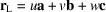, where u, v, w are any integers, denote the position vectors of the point xyz and a lattice point uvw lying in the plane (1.1.2.3), respectively, and assume that r and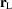are different vectors. If the plane normal is denoted by N, where N is proportional to the vector product of two in-plane lattice vectors, the vector form of the equation of the lattice plane becomes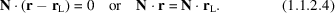For equations (1.1.2.3)and (1.1.2.4)to be identical, the plane normal N must satisfy the requirement that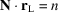, where n is an (unrestricted) integer.

While the Miller indices of lattice planes in P-type lattices must be relatively prime, if the direct lattice is based on a non-primitive unit cell (any centring type) the Miller indices of some lattice planes are no longer relatively prime (e.g. Nespolo, 2015).

Let us now consider the basic diffraction relations (e.g. Lipson & Cochran, 1966). Suppose a parallel beam of monochromatic radiation, of wavelength, falls on a lattice of identical point scatterers. If it is assumed that the scattering is elastic, i.e. there is no change of the wavelength during this process, the wavevectors of the incident and scattered radiation have the same magnitude, which can conveniently be taken as. A consideration of path and phase differences between the waves outgoing from two point scatterers separated by the lattice vector(defined as above) shows that the condition for their maximum constructive interference is given by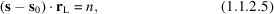whereand s are the wavevectors of the incident and scattered beams, respectively, and n is an arbitrary integer.

Since, where u, v and w are unrestricted integers, equation (1.1.2.5)is equivalent to the equations of Laue:whereis the diffraction vector, and h, k and l are integers corresponding to orders of diffraction from the three-dimensional lattice (Lipson & Cochran, 1966). The diffraction vector thus has to satisfy a condition that is analogous to that imposed on the normal to a lattice plane.

The next relevant aspect to be commented on is the Fourier expansion of a function having the periodicity of the crystal lattice. Such functions are e.g. the electron density, the density of nuclear matter and the electrostatic potential in the crystal, which are the operative definitions of crystal structure in X-ray, neutron and electron-diffraction methods of crystal structure determination. A Fourier expansion of such a periodic function may be thought of as a superposition of waves (e.g. Buerger, 1959), with wavevectors related to the interplanar spacings, in the crystal lattice. Denoting the wavevector of a Fourier wave by g (a function of hkl), the phase of the Fourier wave at the point r in the crystal is given by, and the triple Fourier series corresponding to the expansion of the periodic function, say G(r), can be written aswhere C(g) are the amplitudes of the Fourier waves, or Fourier coefficients, which are related to the experimental data. Numerous examples of such expansions appear throughout this volume.

The permissible wavevectors in the above expansion are restricted by the periodicity of the function G(r). Since, by definition,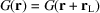, whereis a direct-lattice vector, the right-hand side of (1.1.2.7)must remain unchanged when r is replaced by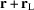. This, however, can be true only if the scalar product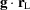is an integer.

Each of the above three aspects of crystallography may lead, independently, to a useful introduction of the reciprocal vectors, and there are many examples of this in the literature. It is interesting, however, to consider the representation of the equation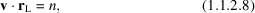which is common to all three, in its most convenient form. Obviously, the vector v which stands for the plane normal, the diffraction vector, and the wavevector in a Fourier expansion, may still be referred to any permissible basis and so may, by an appropriate transformation.

Let, where A, B and C are linearly independent vectors. Equation (1.1.2.8)can then be written asor, in matrix notation,orThe simplest representation of equation (1.1.2.8)results when the matrix of scalar products in (1.1.2.11)reduces to a unit matrix. This can be achieved (i) by choosing the basis vectorsto be orthonormal to the basis vectors, while requiring that the components ofbe integers, or (ii) by requiring that the basesandcoincide with the same orthonormal basis, i.e. expressing both v and, in (1.1.2.8), in the same Cartesian system. If we choose the first alternative, it is seen that:

 (1) The components of the vector v, and hence those of N, h and g, are of necessity integers, since u, v and w are already integral. The components of v include Miller indices in the case of the lattice plane, they coincide with the orders of diffraction from a three-dimensional lattice of scatterers, and correspond to the summation indices in the triple Fourier series (1.1.2.7). (2) The basis vectors A, B and C are reciprocal to a, b and c, as can be seen by comparing the scalar products in (1.1.2.11)with those in (1.1.2.1)and (1.1.2.2). In fact, the basesandare mutually reciprocal. Since there are no restrictions on the integers U, V and W, the vector v belongs to a lattice which, on account of its basis, is called the reciprocal lattice.

It follows that, at least in the present case, algebraic simplicity goes together with ease of interpretation, which certainly accounts for much of the importance of the reciprocal lattice in crystallography. The second alternative of reducing the matrix in (1.1.2.11)to a unit matrix, a transformation of (1.1.2.8)to a Cartesian system, leads to non-integral components of the vectors, which makes any interpretation of v ormuch less transparent. However, transformations to Cartesian systems are often very useful in crystallographic computing and will be discussed below (see also Chapters 2.3and 3.3in this volume).

We shall, in what follows, abandon all the temporary notation used above and write the reciprocal-lattice vector asorand denote the direct-lattice vectors by, as above, or by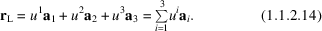The representations (1.1.2.13)and (1.1.2.14)are used in the tensor-algebraic formulation of the relationships between mutually reciprocal bases (see Section 1.1.4below).

References

Buerger, M. J. (1941). X-ray Crystallography. New York: John Wiley.
Buerger, M. J. (1959). Crystal Structure Analysis. New York: John Wiley.
Ewald, P. P. (1913). Zur Theorie der Interferenzen der Röntgenstrahlen in Kristallen. Phys. Z. 14, 465–472.
Ewald, P. P. (1921). Das reziproke Gitter in der Strukturtheorie. Z. Kristallogr. 56, 129–156.
Laue, M. (1914). Die Interferenzerscheinungen an Röntgenstrahlen, hervorgerufen durch das Raumgitter der Kristalle. Jahrb. Radioakt. Elektron. 11, 308–345.
Lipson, H. & Cochran, W. (1966). The Determination of Crystal Structures. London: Bell.
Nespolo, M. (2015). The ash heap of crystallography: restoring forgotten basic knowledge. J. Appl. Cryst. 48, 1290–1298.
Shmueli, U. (2007). Theories and Techniques of Crystal Structure Determination, Section 1.2. Oxford University Press.
Wilson, E. B. (1901). Vector Analysis. New Haven: Yale University Press.